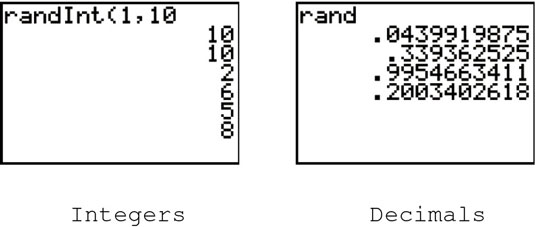##### Ti-84 Plus Graphing Calculator For DummiesYou can use the TI-84 Plus graphing calculator to calculate probabilities such as permutations and combinations and to generate random integers and decimals. Do you need to calculate the number of ways you can arrange six people at a table or the number of ways you can select four people from a group of six people? Or do you just need an unbiased way of selecting people at random?

## Permutations and combinations on the TI-84 Plus

A permutation, denoted by nPr, answers the question: “From a set of n different items, how many ways can you select and order (arrange) r of these items?” A combination, denoted by nCr, answers the question: “From a set of n different items, how many ways can you select (independent or order) r of these items?” To evaluate a permutation or combination, follow these steps:

1. On the Home screen, enter n, the total number of items in the set.

If you’re not already on the Home screen, press [2nd][MODE] to exit (quit) the current screen and enter the Home screen.

2. Press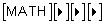to access the Math Probability menu.

3. Press  to evaluate a permutation or press  to evaluate a combination.

4. Enter r, the number of items selected from the set, and press [ENTER] to display the result.

Notice that the last two lines in the figure tell you that it’s impossible to select 7 items from a set of only 5 items.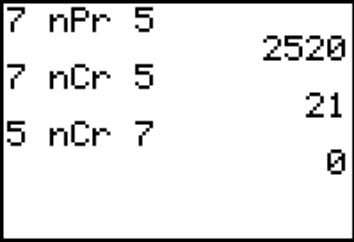## How to generate random numbers on the TI-84 Plus

When generating random numbers, you usually want to generate numbers that are integers contained in a specified range, or decimal numbers that are strictly between 0 and 1.

To generate random integers that fall between the integers a and b:

1. Press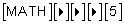to select the randInt command from the Math Probability menu.

2. Enter the value of the lower limit a, press [,], and enter the upper limit b.

3. Press [ENTER] to generate the first random integer. Repeatedly press [ENTER] to generate more random integers.

Pressing any key except [ENTER] stops the calculator from generating random integers.

To generate random decimal numbers that are strictly between 0 and 1, press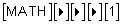to select the rand command from the Math Probability menu. Then repeatedly press [ENTER] to generate the random numbers.